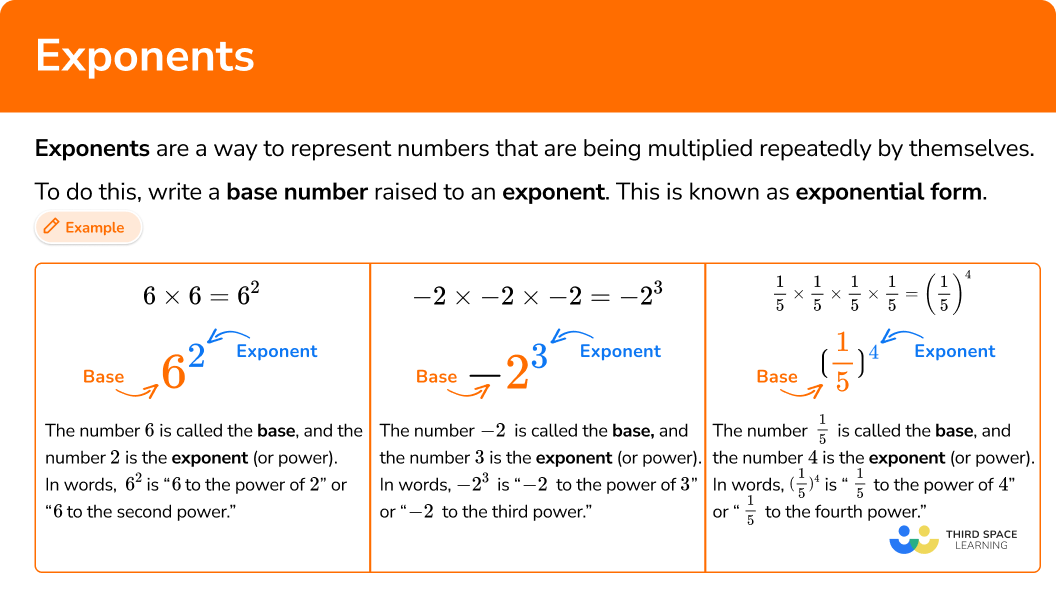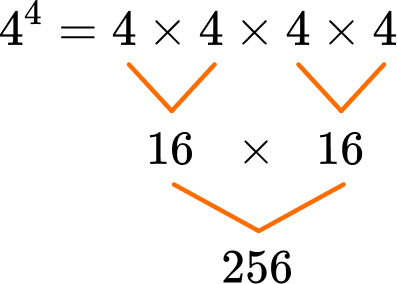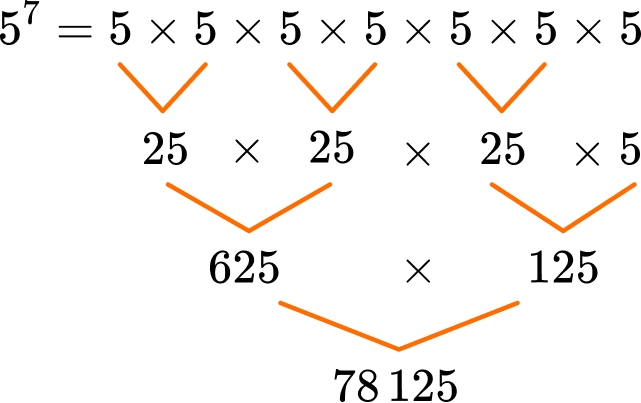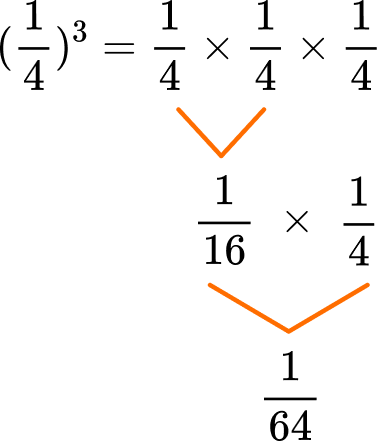# Exponents

Here you will learn about exponents, including what they are and how to write and solve equations using exponents.

Students will first learn about exponents as part of numbers and operations in base ten in 5th grade, and then as part of expressions and equations in 6th grade.

## What are exponents?

Exponents are a way to represent numbers that are being multiplied repeatedly by themselves.

For example,

• You can write 3 \times 3 in a shorter way using exponents: 3^2.
You can say this as 3 to the power of 2.

• You can write -2 \times-2 \times-2 in a shorter way, using exponents -2^3.
You can say this as -2 to the power of 3.

• You can write \cfrac{1}{5} \times \cfrac{1}{5} \times \cfrac{1}{5} \times \cfrac{1}{5} \, in a shorter way, using exponents \left(\cfrac{1}{5}\right)^4.
You can say this as \, \cfrac{1}{5} \, to the power of 4.

This is known as exponential form. In exponential form, you write a base number raised to an exponent. The base number can be an integer, decimal, fraction, etc., and the exponent tells us the number of times you multiply the base number.

For example,

The number 6 is called the base, and the number 2 is the exponent (or power). In words, 6^2 is “ 6 to the power of 2 ” or “ 6 to the second power.”

For exponent 2, you can also say “ 6 squared.” This relates to the area formula for squares: \text { length } \times \text { width}. Since squares always have the same length and width, their area will always be the same number multiplied by itself.

For example, 6 \times 6 or more generally \mathrm{s} \times \mathrm{s}. Because of this, you say the product of the area or the exponent is “squared.”

Exponent 3 also has another name. Let’s look at 4 \times 4 \times 4=4^3.

The number 4 is the base, and the number 3 is the exponent (or power). In words, 4^3 is “ 4 to the power of 3 ” or “ 4 to the third power.”

For exponent 3, you can also say “ 4 cubed.” This relates to the volume formula for cubes: \text { length } \times \text { width } \times \text { height}. Since cubes always have the same length, width, and height, their volume will always be the same number multiplied by itself three times.

Like 4 \times 4 \times 4 or more generally c \times c \times c. Because of this, you say the product of the area or the exponent is “cubed.”

Exponents are also used to represent numbers by powers of \bf{10} (scientific notation).

For example,

4 \times 10^4=4 \times 10,000=40,000

or

6 \div 10^4=6 \div 10,000=0.0006

The power of 10, in this case 10^4 (or 10,000 ), changes the place value of the numbers multiplied or divided by it.

Multiplying by 10^4 shifts the digits 4 places to the left.

Dividing by 10^4 shifts the digits 4 places to the right.

This is the same rule for any power of 10.

Step-by-step guide: Powers of 10

### What are exponents?## Common Core State Standards

How does this relate to 5th and 6th grade math?

• Grade 5 – Numbers and Operations in Base Ten (5.NBT.2)
Explain patterns in the number of zeros of the product when multiplying a number by powers of 10, and explain patterns in the placement of the decimal point when a decimal is multiplied or divided by a power of 10. Use whole-number exponents to denote powers of 10.

• Grade 6 – Expressions and Equations (6.EE.A.1)
Write and evaluate numerical expressions involving whole-number exponents.

## How to use exponents

In order to rewrite an expression with exponents:

1. Identify the base(s).
2. Count the number of times the base is being multiplied.
3. Rewrite the expression.

In order to solve an equation with exponents:

1. Change the equation from exponential form to expanded form.
2. Solve the equation.

## Exponent examples

### Example 1: rewrite an expression with exponents

Rewrite the expression 11 \times 11 \times 11 \times 11 in exponential form.

1. Identify the base(s).

The base is the number being multiplied. The base is 11.

2Count the number of times the base is being multiplied.

11 is being multiplied 4 times. So the exponent will be 4.

3Rewrite the expression.

11 \times 11 \times 11 \times 11=11^4

You read 11^4 as “ 11 to the power of 4 ” or “ 11 to the fourth power.”

### Example 2: rewrite an expression with exponents

Rewrite the expression 3 \times 3 \times 2 \times 3 \times 3 \times 3 in exponential form.

The bases are the numbers being multiplied. The bases are 3 and 2.

The number 2 is multiplied just once, so you can write it as 2^1, but it does not need to be written in exponential form.

3 is being multiplied 5 times. So the exponent will be 5.

3 \times 3 \times 2 \times 3 \times 3 \times 3=3^5 \times 2

You read 3^5 as “ 3 to the power of 5 ” or “ 3 to the fifth power.”

### Example 3: rewrite an expression including fractions with exponents

Rewrite the expression \, \cfrac{1}{2} \times \cfrac{1}{2} \times \cfrac{1}{2} \times 7 \times 7 in exponential form.

The bases are the numbers being multiplied. The bases are \, \cfrac{1}{2} \, and 7.

\cfrac{1}{2} \, is being multiplied 3 times. So the exponent will be 3.

7 is being multiplied 2 times. So the exponent will be 2.

\cfrac{1}{2} \times \cfrac{1}{2} \times \cfrac{1}{2} \times 7 \times 7=\left(\cfrac{1}{2}\right)^3 \times 7^2

You read \, \left(\cfrac{1}{2}\right)^3 as “ \, \cfrac{1}{2} \, to the power of 3, ” “ \, \cfrac{1}{2} \, cubed” or “ \, \cfrac{1}{2} \, to the third power.”

You read 7^2 as “ 7 to the power of 2, ” “ 7 squared” or “ 7 to the second power.”

### Example 4: solve an equation with exponents

Solve 6^4 = \, ?

The base is the number being multiplied. The base is 6.

The exponent tells us how many times the base is multiplied. The exponent is 4.

6^4=6 \times 6 \times 6 \times 6

So, 6^4=6 \times 6 \times 6 \times 6=1,296.

### Example 5: solve an equation with exponents

Solve 5^2 \times 4^3 = \, ?

The bases are the numbers being multiplied. The bases are 5 and 4.

The exponent tells us how many times the base is multiplied. The exponents are 2 and 3.

\begin{aligned} & 5^2=5 \times 5 \\\\ & 4^3=4 \times 4 \times 4 \end{aligned}

So, 5^2 \times 4^3=5 \times 5 \times 4 \times 4 \times 4=1,600.

### Example 6: solve an equation including fractions with exponents

Solve \, \left(\cfrac{2}{3}\right)^5 = \, ?

The base is the number being multiplied. The base is \, \cfrac{2}{3}.

The exponent tells us how many times the base is multiplied. The exponent is 5.

\left(\cfrac{2}{3}\right)^5=\cfrac{2}{3} \times \cfrac{2}{3} \times \cfrac{2}{3} \times \cfrac{2}{3} \times \cfrac{2}{3}

So \, \left(\cfrac{2}{3}\right)^5=\cfrac{2}{3} \times \cfrac{2}{3} \times \cfrac{2}{3} \times \cfrac{2}{3} \times \cfrac{2}{3}=\cfrac{32}{243}.

### Teaching tips for exponents

• Start with less complicated exponential expressions when first introducing this topic. Sticking to whole numbers and exponents 5 and under will allow students to focus on understanding exponents rather than being caught up in calculations. Once students have a good grasp of exponents at this level, then move forward to use rational numbers as the base and exponents larger than 5.

• Worksheets are a good way to provide students with practice, but consider also letting students solve in other ways. There are interactive websites and games or something as simple as letting students practice on white boards can help make practicing more engaging.

• Have students discuss why exponents, and other shortcut symbols in math, are useful and allow them to conjecture why the symbols might have been created in the first place. You can start by saying something like “Imagine if we used only words to represent math. For example, if we had to write down ‘two plus two equals 4 ’ in words instead of using symbols. How would that impact our ability to do math? Why are symbols and other math shortcuts like exponents useful?”

### Easy mistakes to make

• Multiplying the base and the exponent
The exponent tells us how many times to multiply the base number by itself.
You do not multiply the base by the exponent.
For example,

• Miscounting the times a base number is multiplied
In order to write an expression in exponential form, you need to count the number of times a base is being multiplied. It is easy to miscount with expressions that contain many different numbers.
For example,
^The base 4 was miscounted
• Forgetting about an exponent of \bf{1}
Every number is technically itself to the power of 1. It is not typically written, but remember that it’s there.
For example,
\begin{aligned} &8=8^1 \\ &\cfrac{1}{8}=\left(\cfrac{1}{8}\right)^1 \\ &1.8=1.8^1 \end{aligned}

### Practice to the power of questions

1. Solve 5^2 = \, ?

10251532The base is the number being multiplied. The base is 5.

The exponent tells us how many times the base is multiplied. The exponent is 2.

5^2=5 \times 5=25

2. Write 2 \times 7 \times 7 \times 2 \times 7 \times 7 in exponential form.

2^2 \times 7^42 \times 7^22^4 \times 7(2 \times 7)^6The bases are the numbers being multiplied. The bases are 2 and 7.

2 is being multiplied 2 times. So the exponent will be 2.

7 is being multiplied 4 times. So the exponent will be 4.

2^2 \times 7^4

You read 2^2 as “ 2 to the power of 2, ” “ 2 squared” or “ 2 to the second power.”

You read 7^4 as “ 7 to the power of 4 ” or “ 7 to the fourth power.”

3. Solve 4^4 = \, ?

1664256356The base is the number being multiplied. The base is 4.

The exponent tells us how many times the base is multiplied. The exponent is 4.So, 4^4=4 \times 4 \times 4 \times 4=256

4. Solve 5^7 = \, ?

78,12515,62516,80735The base is the number being multiplied. The base is 5.

The exponent tells us how many times the base is multiplied. The exponent is 7.5^7=5 \times 5 \times 5 \times 5 \times 5 \times 5 \times 5=78,125

5. Write 0.3 \times 3 \times 0.3 \times 0.3 \times 0.3 in exponential form.

3^4 \times 0.3(0.3 \times 3)^50.3^4 \times 30.3 \times 3^4The bases are the numbers being multiplied. The bases are 0.3 and 3.

The number 3 is multiplied just once, so we can write it as 3^1, but it does not need to be written in exponential form.

0.3 is being multiplied 4 times. So the exponent will be 4.

0.3 \times 3 \times 0.3 \times 0.3 \times 0.3=0.3^4 \times 3

You read 0.3^4 as “ 0.3 to the power of 4 ” or “ 0.3 to the fourth power.”

6. Solve \, \left(\cfrac{1}{4}\right)^3

\cfrac{3}{4}\cfrac{1}{12}\cfrac{1}{64}\cfrac{4}{64}The base is the number being multiplied. The base is \, \cfrac{1}{4}.

The exponent tells us how many times the base is multiplied. The exponent is 3.So, \left(\cfrac{1}{4}\right)^3=\cfrac{1}{4} \times \cfrac{1}{4} \times \cfrac{1}{4}=\cfrac{1}{64}

## Exponents FAQs

Why do you include parentheses when the base is a fraction?

This ensures that the exponent is applied to both the numerator and the denominator. Otherwise, the exponent may only apply to the numerator.

Are there other types of exponents besides positive exponents?

Yes, there are fractional exponents, where the exponent is a fraction.
For example, \, 4^{\frac{1}{2}}=2. There are also negative exponents, where the exponent is a negative number. For example, \, ​​3^{-2}=\cfrac{1}{3^2}. These are covered in later grades.

When the product is a fraction, do you have to simplify it?

No, you do not have to answer in simplified form. However, it is a best practice and should be done when the directions or state standards specify.

When do students learn the rules of exponents (laws of exponents)?

This comes later in middle school and continues into high school. By the end of high school students will be able to apply exponents to all real numbers and polynomials.

What happens as the exponent of a number increases?

Each time an exponent is added the number grows exponential. This is best seen by graphing an exponential equation.

## Still stuck?

At Third Space Learning, we specialize in helping teachers and school leaders to provide personalized math support for more of their students through high-quality, online one-on-one math tutoring delivered by subject experts.

Each week, our tutors support thousands of students who are at risk of not meeting their grade-level expectations, and help accelerate their progress and boost their confidence.